Index

Working with MUMIE as author

Working with MUMIE as teacher

Using MUMIE via plugin in local LMS

FAQ for examination lecturers

Overview

Go back to the old wiki

We revise and update this wiki. We apologize for the inconvenience this may cause you.

# MC Score Types

For an answer of type mc.matrix or mc.multiple (and partially mc.yesno) you can not only set the score factor with \score but also choose between different manners for computing the score:

a) Type AllMustBeCorrect If you want the students only geting points if all choices are correct, use \allMustBeCorrect. (works for type mc.yesno)
b) For other Types use the command: \MCScoreType{<score type>} (does not yet work for type mc.yesno)

score type How the score is computed mc.multiple mc.yesno mc.matrix
1 max(0, (TP - (FP / (N + 1) ) ) / P) ✔️ (default) ✔️ (default)
2 (TP + TN) / (P + N) ✔️ ✔️
3 max(0, (TP - FP) / P) ✔️ ✔️
4 column-wise (correct columns / number of columns) ✔️
5 1 if FP + FN = 0; 0.5 if FP + FN = 1; 0 otherwise (Kprim) ✔️ ✔️ ✔️
6 TP/P if FP = 0; 0 otherwise ✔️ ✔️
7 max(0, ((TP + TN) - (FP + FN)) / (P + N)) ✔️ ✔️ ✔️
8 row-wise (correct rows / number of rows) ✔️
9 1 if TP > 0 and FP = 0; 0 otherwise ✔️
??? further types will be available in the future ? ? ?

TP: true positive, FP: false positive, TN: true negative, FN: false negative, P: number of positive answers, N: number of negative answers

 student answer ☑ student answer ☐ expected ☑ TP FN expected ☐ FP TN

Remark: The commands \score and \MCScoreType are only available within an answer, but not on question level.

Example: Select all true statements

Type 1 Type 2 Type 3 Type 5 Type 6 Type 7 Type 9 \allMustBeCorrect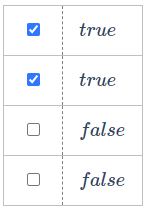P=N=2

TP=TN=2
FP=FN=0
100% 100% 100% 100% 100% 100% 100% 100%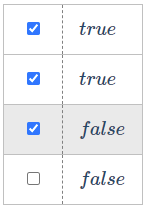P=N=2

TP=2, TN=1
FP=1, FN=0
83,33333% 75% 50% 50% 0% 50% 0% 0%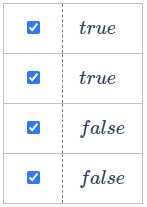P=N=2

TP=2, TN=0
FP=2, FN=0
66,66667% 50% 0% 0% 0% 0% 0% 0%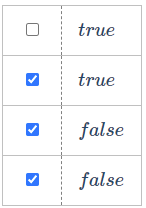P=N=2

TP=1, TN=0
FP=2, FN=1
16,666667% 25% 0% 0% 0% 0% 0% 0%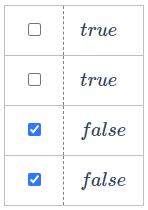P=N=2

TP=TN=0
FP=FN=2
0% 0% 0% 0% 0% 0% 0% 0%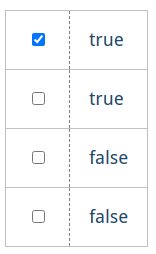P=N=2

TP=1, TN=2
FP=0, FN=1
50% 75% 50% 50% 50% 50% 100% 0%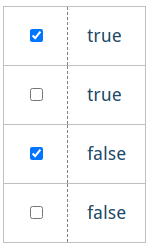P=N=2

TP=TN=1
FP=FN=1
33.333334% 50% 0% 0% 0% 0% 0% 0%
1234567891011121314151617181920212223242526272829303132333435363738394041424344454647484950515253545556575859\begin{answer}       \type{mc.matrix}        \text{...}        \tasks{...}         \score{6}        \MCScoreType{2}         \begin{row}        % ...        \end{row}         \begin{row}            % ...        \end{row} \end{answer} \begin{answer}       \type{mc.matrix}        \text{...}        \tasks{...}         \score{6}        \allMustBeCorrect         \begin{row}        % ...        \end{row}         \begin{row}            % ...        \end{row} \end{answer} \begin{answer}       \type{mc.multiple}        \text{...}         \score{4}        \MCScoreType{3}      % ... \end{answer} \begin{answer}       \type{mc.multiple}        \text{...}         \score{4}        \allMustBeCorrect      % ... \end{answer}